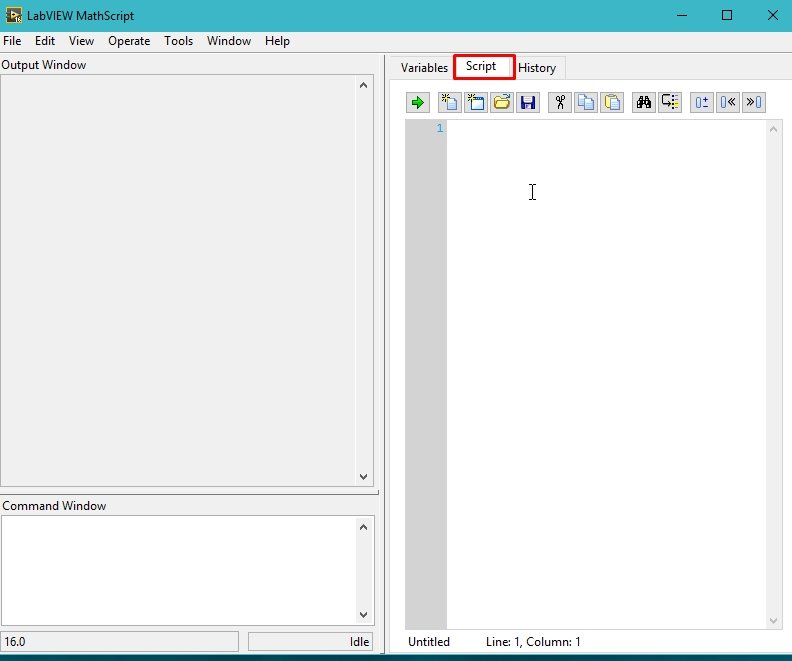# How to use MathScripts in LabView : tutorial 15

In this tutorial, I have explained the working of MathScript in LabView. At the start an explanatory introduction of MathScript is provided to have some basic knowledge of the window. After that some of the basic commands and their uses are explained with examples. MathScript is a very extensive programming environment itself, it is impossible to explain its entire feature in one tutorial. However I tried to explain the basic features and gave you some knowledge of how to do self study on MathScript. At the end of the tutorial you are provided with an exercise to do it by yourself, and in the next tutorials I will assume that you have done those exercises and I will not explain the concept regarding them.

### Introduction to mathScripts in labview

Textual math can be added to the LabView development environment with a compiler for the .m files, added by LabView MathScript Modules. Textual and graphical approaches can be blend together in MathScript. It may be used for algorithm development, control designs, also including data processing and analysis and signal processing tasks. Without extra code generation steps, you can easily deploy your .m code to real-time hardware.

It has many advantages over different programming languages i.e. you can save you valuable time with LabVIEW MathScript, which is generally compatible with almost all the .m file compilers i.e. MATLAB and Octave etc. You can reuse your already developed i.e. existing .m files in line with G code for analysis, processing or data presentation using this module of LabView.

Libraries related to engineering and scientific control like dials and controls are already present in the built-in LabVIEW GUI. You can always use the built-in LabView features to add user interaction and decision making to your .m files, and take advantages from LabViews’ MathScript.

You have all of the tools you need to deploy your custom .m files for deterministic execution with LabVIEW and MathScript, with a framework to both develop and test your .m files.

### Explanation MathScripts with example

Figure 1: Main page

Figure 2: Opening MathScript window

Figure 3: Initializing a mathscript window

Figure 4: Help instruction

Figure 5: Help classes

Figure 6: Help window

Figure 7: Features of help window

Figure 8: Variables portion

• All variables once declared or used, either in command window or in the script will appear in this section, no matter either the variables used in the code are local or global.
• The code written in mathscript cannot be accessed again as it is not saved nowhere. However we can write a code in mathscript that can be saved.
• The script to write multiple lines of code and save it for future use is also available in mathscript window. The figure below shows a blank script in which you can write desired code.Figure 9: Script

Figure 10: History

Figure 12: Suppressed output

Figure 13: Array declaration

Figure 14: Array transpose

Figure 15: Matrix multiplication error

Figure 16: Satisfied matrix multiplication

Figure 17: Matrix in variable window

Figure 18: Script code

Figure 19: Saving a script

Figure 20: Running the script

Figure 21: Output of script

Figure 22: Present working directory.

Figure 23: Multiple point generation

Figure 24: Multipoint output

Figure 25: Sin function generation

Figure 26: Output of sin

The resolution of the sin function can be increased by decreasing the interval of the t function from 0.01 to 0.0001 or further less.

Exercise:

• Plot all the trigonometric functions that are feasible for plotting.
• Try to adjust the range of x and y axis
• Label both the axes and give title to your graphs.

(Hint: Use help command for all the above tasks)

<< Previous tutorial                                Next tutorial>>

### 1 thought on “How to use MathScripts in LabView : tutorial 15”

1. The best site to learn LabVIEW. Thanks a lot.